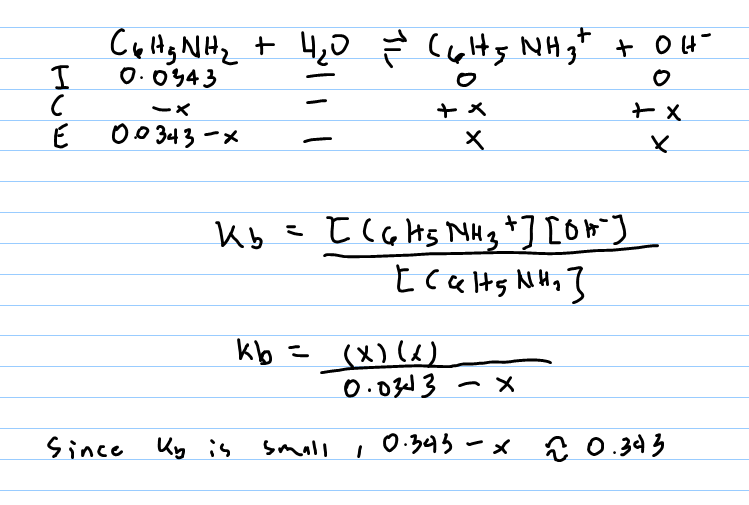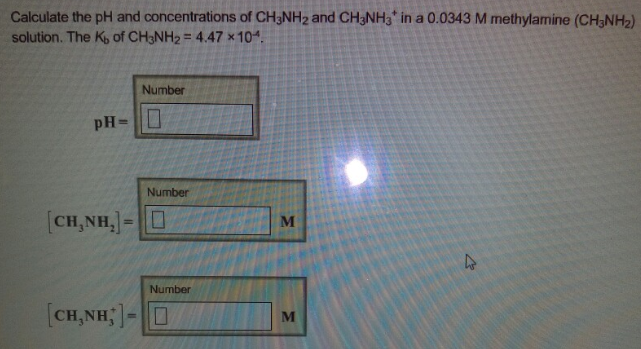# Problem: Calculate the pH and concentrations of CH3NH2 and CH3NH3+ in a 0.0343 M methylamine (CH3NH2) solution. The Kb of CH3NH2 = 4.47 x 10-4. pH= [CH3NH2] = [CH3NH3+] =

###### FREE Expert Solution100% (213 ratings)###### Problem Details

Calculate the pH and concentrations of CH3NH2 and CH3NH3+ in a 0.0343 M methylamine (CH3NH2) solution. The Kb of CH3NH2 = 4.47 x 10-4

pH=

[CH3NH2] =

[CH3NH3+] =